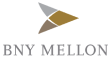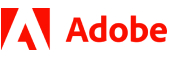New update is available. Click here to update.
Topics

# Complete String

Hard0/120
Average time to solve is 40m+4 more companies

## Problem statement

Ninja developed a love for arrays and strings so this time his teacher gave him an array of strings, ‘A’ of size ‘N’. Each element of this array is a string. The teacher taught Ninja about prefixes in the past, so he wants to test his knowledge.

A string is called a complete string if every prefix of this string is also present in the array ‘A’. Ninja is challenged to find the longest complete string in the array ‘A’.If there are multiple strings with the same length, return the lexicographically smallest one and if no string exists, return "None".

Note :
``````String ‘P’ is lexicographically smaller than string ‘Q’, if :

1. There exists some index ‘i’ such that for all ‘j’ < ‘i’ , ‘P[j] = Q[j]’ and ‘P[i] < Q[i]’. E.g. “ninja” < “noder”.

2. If ‘P’ is a prefix of string ‘Q’, e.g. “code” < “coder”.
``````
Example :
``````N = 4
A = [ “ab” , “abc” , “a” , “bp” ]

Explanation :

Only prefix of the string “a” is “a” which is present in array ‘A’. So, it is one of the possible strings.

Prefixes of the string “ab” are “a” and “ab” both of which are present in array ‘A’. So, it is one of the possible strings.

Prefixes of the string “bp” are “b” and “bp”. “b” is not present in array ‘A’. So, it cannot be a valid string.

Prefixes of the string “abc” are “a”,“ab” and “abc” all of which are present in array ‘A’. So, it is one of the possible strings.

We need to find the maximum length string, so “abc” is the required string.
``````
Detailed explanation ( Input/output format, Notes, Images )
Constraints :
``````1 <= T <= 10
1 <= N <= 10^5
1 <= A[i].length <= 10^5
A[i] only consists of lowercase english letters.
Sum of A[i].length <= 10^5 over all test cases

Time Limit : 1 sec
``````
Sample Input 1 :
``````2
6
n ni nin ninj ninja ninga
2
ab bc
``````
Sample Output 1 :
``````ninja
None
``````
Explanation Of Sample Input 1 :
``````For test case 1 we have,

All the prefixes of “ninja” -> “n”, “ni”, “nin”, “ninj” and “ninja” are present in array ‘A’. So, “ninja” is a valid answer whereas for “ninga” , the prefix “ning” is not present in array ‘A’.

So we output “ninja”.

For test case 2 we have,

The prefixes of “ab” are “a” and “ab”. “a” is not present in array ‘A’. So, “ab” is not a valid answer.

The prefixes of “bc” are “b” and “bc”. “b” is not present in array ‘A’. So, “ab” is not a valid answer.

Since none of the strings is a valid answer we output “None”.
``````
Sample Input 2 :
``````2
5
g a ak szhkb hy
4
kez vfj vfjq vfjqo
``````
Sample Output 2 :
``````ak
None
``````Console